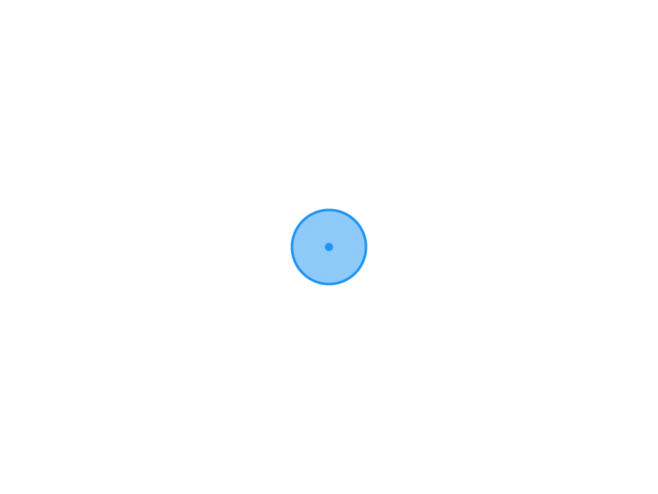TypechoJoeTheme

# 日志随记

/XG.孤梦

# C语言循环结构与循环嵌套-学习十2022-02-01
/
0 评论
/
623 阅读
/
387 个字
/

02/01

## break语句和continue语句

• break语句提前终止循环

• break语句可以用来从循环体内跳出循环体，即提前结束循环，接着执行循环下面的语句。
• 只能用于循环语句和switch语句之中，而不能单独使用
• continue语句提前结束本次循环

• 有时并不希望终止整个循环的操作,只希望提前结束本次循环，而接着执行下次循环，这时可以用continue语句。
• break语可和continue语句的区别

• continue语句只结束本次循环,而不是终止整个循环的执行。
• break语句结束整个循环过程,不再判断执行循环的条件是否成立。

#### 例子

``````#include <stdio.h>
#define SUM 100000

void main() {
float amount, aver, total, i;

for (i=1,total=0;i<= 1000;i++)
{
printf("请输入数额：");
scanf_s("%f", &amount);
total = amount + total;
if (total >= SUM)
break;
}
aver = total / i;

printf("平均：%f\n", aver);
}````````````#include <stdio.h>

void main() {
int i;

for (i=100;i<= 200;i++)
{
if (i % 3 != 0)
printf("%d, ", i);
else
continue;
}
}``````## 循环嵌套

• 一个循环体内又包含另一个完整的循环结构,称为循环的嵌套
• 内嵌的循环中还可以嵌套循环，这就是多层循环
• 3种循环(while循环、do...while循环和for循环)可以互相嵌套

#### 例子

，键盘输入打印行数，输出下列图案

``````     *
**
***
****
*****
...``````
``````#include <stdio.h>

void main() {
int i,j,n;
printf("请输入打印的行数：");
scanf_s("%d", &n);
for (i=1;i<= n;i++)
{
for (j = 1; j <= n - i; j++)
printf(" ");
for (j = 1; j <= i; j++)
printf("*");
printf("\n");
}
}
````````````#include <stdio.h>
#include <stdlib.h>
#include <time.h>

void main() {
srand(time(0)); //根据系统时间设置随机数种
int i,j,n =0;
for (i=1;i<= 4;i++)
{
for (j = 1; j <= 5; j++)
printf("%d\t",rand()% 100);  //取得区间[O,100)的随机整数
printf("\n");
}
}
``````https://www.xggm.top/archives/292.html（转载时请注明本文出处及文章链接）XG.孤梦博 主大佬

51 文章数
102 评论量

X